# Python Built-in Functions

## Python Programming Training Certification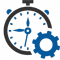Flexible Hours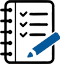100 Assignments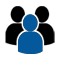Instructor Led online Training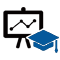50 LMS Access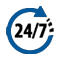24X7 Support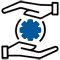100% Skill Level

### Enquire Now

4.9 out of 1000+ Ratings
Best Python Institute for Learning Python Course & Training, Live Project Training in Python with Django, Data Science and AI, Interview Assistance, Expert Coaching Trainers. Python Certification & Interview Assistance! Get free demo now!

### Course Overview

Python is one of the world’s top programming languages used today and Python training has become the most popular training across individuals. Training Basket’s Python Training & Certification course covers basic and advanced Python concepts and how to apply them in real-world applications.Python is a flexible and powerful open-source language that is easy to learn and consists of powerful libraries for data analysis and manipulation. Our Python training course content is curated by experts as per the standard Industry curriculum. The curriculum, coding challenges and real-life problems cover data operations in Python, strings, conditional statements, error handling, shell scripting, web scraping and the commonly used Python web framework Django. Take this Python training and certification course and become job-ready now.### Python Function

The Python built-in functions are defined as the functions whose functionality is pre-defined in Python. The python interpreter has several functions that are always present for use. These functions are known as Built-in Functions. There are several built-in functions in Python which are listed below:
Python abs() Function

The pythonabs()function is used to return the absolute value of a number. It takes only one argument, a number whose absolute value is to be returned. The argument can be an integer and floating-point number. If the argument is a complex number, then, abs() returns its magnitude.
Python abs() Function Example

• # integer number
integer = -20
print(‘Absolute value of -40 is:’, abs(integer))

# floating number
floating = -20.83
print(‘Absolute value of -40.83 is:’, abs(floating))

Output:

• Absolute value of -20 is: 20
Absolute value of -20.83 is: 20.83

Python all() FunctionThe pythonall()function accepts an iterable object (such as list, dictionary, etc.). It returns true if all items in passed iterable are true. Otherwise, it returns False. If the iterable object is empty, the all() function returns True.Python all() Function Example

• # all values true
k = [1, 3, 4, 6]
print(all(k))

# all values false
k = [0, False]
print(all(k))

# one false value
k = [1, 3, 7, 0]
print(all(k))

# one true value
k = [0, False, 5]
print(all(k))

# empty iterable
k = []
print(all(k))

Output:

• True
False
False
False
True

Python bin() FunctionThe pythonbin()function is used to return the binary representation of a specified integer. A result always starts with the prefix 0b.Python bin() Function Example

• x = 10
y = bin(x)
print (y)

Output:

• 0b1010

Python bool()

The pythonbool()converts a value to boolean(True or False) using the standard truth testing procedure.
Python bool() Example

• test1 = []
print(test1,’is’,bool(test1))
test1 = 
print(test1,’is’,bool(test1))
test1 = 0.0
print(test1,’is’,bool(test1))
test1 = None
print(test1,’is’,bool(test1))
test1 = True
print(test1,’is’,bool(test1))
test1 = ‘Easy string’

Output:

• [] is False
 is True
0.0 is False
None is False
True is True
Easy string is True

Python bytes()

The pythonbytes()in Python is used for returning abytesobject. It is an immutable version of the bytearray() function.
It can create empty bytes object of the specified size.
Python bytes() Example

• string = “Hello World.”
array = bytes(string, ‘utf-8’)
print(array)

Output:

• b ‘ Hello World.’

Python callable() Function

A pythoncallable()function in Python is something that can be called. This built-in function checks and returns true if the object passed appears to be callable, otherwise false.
Python callable() Function Example

• x = 8
print(callable(x))

Output:

• False

Python compile() FunctionThe pythoncompile()function takes source code as input and returns a code object which can later be executed by exec() function.Python compile() Function Example

• # compile string source to code
code_str = ‘x=5\ny=10\nprint(“sum =”,x+y)’
code = compile(code_str, ‘sum.py’, ‘exec’)
print(type(code))
exec(code)
exec(x)

Output:

• <class ‘code’>
sum = 15

Python exec() Function
The pythonexec()function is used for the dynamic execution of Python program which can either be a string or object code and it accepts large blocks of code, unlike the eval() function which only accepts a single expression.Python exec() Function Example

• x = 8
exec(‘print(x==8)’)
exec(‘print(x+4)’)

Output:

• True
12

Python sum() FunctionAs the name says, pythonsum()function is used to get the sum of numbers of an iterable, i.e., list.Python sum() Function Example

• s = sum([1, 2,4 ])
print(s)

s = sum([1, 2, 4], 10)
print(s)

Output:

• 7
17

Python any() FunctionThe pythonany()function returns true if any item in an iterable is true. Otherwise, it returns False.Python any() Function Example

• l = [4, 3, 2, 0]
print(any(l))

l = [0, False]
print(any(l))

l = [0, False, 5]
print(any(l))

l = []
print(any(l))

Output:

• True
False
True
False

Python ascii() FunctionThe pythonascii()function returns a string containing a printable representation of an object and escapes the non-ASCII characters in the string using \x, \u or \U escapes.Python ascii() Function Example

• normalText = ‘Python is interesting’
print(ascii(normalText))

otherText = ‘Pythön is interesting’
print(ascii(otherText))

print(‘Pyth\xf6n is interesting’)

Output:

• ‘Python is interesting’
‘Pyth\xf6n is interesting’
Pythön is interesting

Python bytearray() The pythonbytearray()returns a bytearray object and can convert objects into bytearray objects, or create an empty bytearray object of the specified size.Python bytearray() Example

• string = “Python is a programming language.”# string with encoding ‘utf-8’
arr = bytearray(string, ‘utf-8’)
print(arr)

Output:

• bytearray(b’Python is a programming language.’)

Python eval() FunctionThe pythoneval()function parses the expression passed to it and runs python expression(code) within the program.Python eval() Function Example

• x = 8
print(eval(‘x + 1’))

Output:

• 9

Python float()The pythonfloat()function returns a floating-point number from a number or string.Python float() Example

• # for integers
print(float(9))

# for floats
print(float(8.19))

# for string floats
print(float(“-24.27″))

# for string floats with whitespaces
print(float(” -17.19\n”))

# string float error
print(float(“xyz”))

Output:

• 9.0
8.19
-24.27
-17.19
ValueError: could not convert string to float: ‘xyz’

Python format() FunctionThe pythonformat()function returns a formatted representation of the given value.Python format() Function Example

• # d, f and b are a type# integer
print(format(123, “d”))

# float arguments
print(format(123.4567898, “f”))

# binary format
print(format(12, “b”))

Output:

• 123
123.456790
1100

Python frozenset()The pythonfrozenset()function returns an immutable frozenset object initialized with elements from the given iterable.Python frozenset() Example

• # tuple of letters
letters = (‘m’, ‘r’, ‘o’, ‘t’, ‘s’)

fSet = frozenset(letters)
print(‘Frozen set is:’, fSet)
print(‘Empty frozen set is:’, frozenset())

Output:

• Frozen set is: frozenset({‘o’, ‘m’, ‘s’, ‘r’, ‘t’})
Empty frozen set is: frozenset()

Python getattr() FunctionThe pythongetattr()function returns the value of a named attribute of an object. If it is not found, it returns the default value.Python getattr() Function Example

• class Details:
age = 22
name = “Phill”

details = Details()
print(‘The age is:’, getattr(details, “age”))
print(‘The age is:’, details.age)

Output:

• The age is: 22
The age is: 22

Python globals() FunctionThe pythonglobals()function returns the dictionary of the current global symbol table.
ASymbol tableis defined as a data structure which contains all the necessary information about the program. It includes variable names, methods, classes, etc.Python globals() Function Example

• age = 22globals()[‘age’] = 22
print(‘The age is:’, age)

Output:

• The age is: 22

Python hasattr() Function The pythonany()function returns true if any item in an iterable is true, otherwise it returns False.Python hasattr() Function Example

• l = [4, 3, 2, 0]
print(any(l))

l = [0, False]
print(any(l))

l = [0, False, 5]
print(any(l))

l = []
print(any(l))

Output:

• True
False
True
False

Python iter() Function The pythoniter()function is used to return an iterator object. It creates an object which can be iterated one element at a time.Python iter() Function Example

• # list of numbers
list = [1,2,3,4,5]

listIter = iter(list)

# prints ‘1’
print(next(listIter))

# prints ‘2’
print(next(listIter))

# prints ‘3’
print(next(listIter))

# prints ‘4’
print(next(listIter))

# prints ‘5’
print(next(listIter))

Output:

• 1
2
3
4
5

Python len() Function The pythonlen()function is used to return the length (the number of items) of an object.Python len() Function Example

• strA = ‘Python’
print(len(strA))

Output:

• 6

Python list() The pythonlist()creates a list in python.Python list() Example

• # empty list
print(list())

# string
String = ‘abcde’
print(list(String))

# tuple
Tuple = (1,2,3,4,5)
print(list(Tuple))
# list
List = [1,2,3,4,5]
print(list(List))

Output:

• []
[‘a’, ‘b’, ‘c’, ‘d’, ‘e’]
[1,2,3,4,5]
[1,2,3,4,5]

Python locals() Function The pythonlocals()method updates and returns the dictionary of the current local symbol table.
ASymbol tableis defined as a data structure which contains all the necessary information about the program. It includes variable names, methods, classes, etc.Python locals() Function Example

• def localsAbsent():
return locals()

def localsPresent():
present = True
return locals()

print(‘localsNotPresent:’, localsAbsent())
print(‘localsPresent:’, localsPresent())

Output:

• localsAbsent: {}
localsPresent: {‘present’: True}

Python map() Function The pythonmap()function is used to return a list of results after applying a given function to each item of an iterable(list, tuple etc.).Python map() Function Example

return n+n

numbers = (1, 2, 3, 4)
print(result)

# converting map object to set

Output:

• <map object at 0x7fb04a6bec18>
{8, 2, 4, 6}

Python memoryview() Function The pythonmemoryview()function returns a memoryview object of the given argument.Python memoryview () Function Example

• #A random bytearray
randomByteArray = bytearray(‘ABC’, ‘utf-8’)

mv = memoryview(randomByteArray)

# access the memory view’s zeroth index
print(mv)

# It create byte from memory view
print(bytes(mv[0:2]))

# It create list from memory view
print(list(mv[0:3]))

Output:

• 65
b’AB’
[65, 66, 67]

Python object() The pythonobject()returns an empty object. It is a base for all the classes and holds the built-in properties and methods which are default for all the classes.Python object() Example

• python = object()print(type(python))
print(dir(python))

Output:

• <class ‘object’>
[‘__class__’, ‘__delattr__’, ‘__dir__’, ‘__doc__’, ‘__eq__’, ‘__format__’, ‘__ge__’,
‘__getattribute__’, ‘__gt__’, ‘__hash__’, ‘__init__’, ‘__le__’, ‘__lt__’, ‘__ne__’,
‘__new__’, ‘__reduce__’, ‘__reduce_ex__’, ‘__repr__’, ‘__setattr__’, ‘__sizeof__’,
‘__str__’, ‘__subclasshook__’]

Python open() Function The pythonopen()function opens the file and returns a corresponding file object.Python open() Function Example

• # opens python.text file of the current directory
f = open(“python.txt”)
# specifying full path

Output:

• Since the mode is omitted, the file is opened in ‘r’ mode; opens for reading.

Python chr() Function Pythonchr()function is used to get a string representing a character which points to a Unicode code integer. For example, chr(97) returns the string ‘a’. This function takes an integer argument and throws an error if it exceeds the specified range. The standard range of the argument is from 0 to 1,114,111.Python chr() Function Example

• # Calling function
result = chr(102) # It returns string representation of a char
result2 = chr(112)
# Displaying result
print(result)
print(result2)
# Verify, is it string type?
print(“is it string type:”, type(result) is str)

Output:

• ValueError: chr() arg not in range(0x110000)

Python complex() Pythoncomplex()function is used to convert numbers or string into a complex number. This method takes two optional parameters and returns a complex number. The first parameter is called a real and second as imaginary parts.Python complex() Example

• # Python complex() function example
# Calling function
a = complex(1) # Passing single parameter
b = complex(1,2) # Passing both parameters
# Displaying result
print(a)
print(b)

Output:

• (1.5+0j)
(1.5+2.2j)

Python delattr() Function Pythondelattr()function is used to delete an attribute from a class. It takes two parameters, first is an object of the class and second is an attribute which we want to delete. After deleting the attribute, it no longer available in the class and throws an error if try to call it using the class object.Python delattr() Function Example

• class Student:
id = 101
name = “Pranshu”
email = “pranshu@abc.com”
# Declaring function
def getinfo(self):
print(self.id, self.name, self.email)
s = Student()
s.getinfo()
delattr(Student,’course’) # Removing attribute which is not available
s.getinfo() # error: throws an error

Output:

• 101 Pranshu pranshu@abc.com
AttributeError: course

Python dir() Function Pythondir()function returns the list of names in the current local scope. If the object on which method is called has a method named __dir__(), this method will be called and must return the list of attributes. It takes a single object type argument.Python dir() Function Example

• # Calling function
att = dir()
# Displaying result
print(att)

Output:

• [‘__annotations__’, ‘__builtins__’, ‘__cached__’, ‘__doc__’, ‘__file__’, ‘__loader__’,
‘__name__’, ‘__package__’, ‘__spec__’]

Python divmod() FunctionPythondivmod()function is used to get remainder and quotient of two numbers. This function takes two numeric arguments and returns a tuple. Both arguments are required and numericPython divmod() Function Example

• # Python divmod() function example
# Calling function
result = divmod(10,2)
# Displaying result
print(result)

Output:

• (5, 0)

Python enumerate() FunctionPythonenumerate()function returns an enumerated object. It takes two parameters, first is a sequence of elements and the second is the start index of the sequence. We can get the elements in sequence either through a loop or next() method.Python enumerate() Function Example

• # Calling function
result = enumerate([1,2,3])
# Displaying result
print(result)
print(list(result))

Output:

• <enumerate object at 0x7ff641093d80>
[(0, 1), (1, 2), (2, 3)]

Python dict()Pythondict()function is a constructor which creates a dictionary. Python dictionary provides three different constructors to create a dictionary:

• If no argument is passed, it creates an empty dictionary.
• If a positional argument is given, a dictionary is created with the same key-value pairs. Otherwise, pass an iterable object.
• If keyword arguments are given, the keyword arguments and their values are added to the dictionary created from the positional argument.

Python dict() Example

• # Calling function
result = dict() # returns an empty dictionary
result2 = dict(a=1,b=2)
# Displaying result
print(result)
print(result2)

Output:

• {}
{‘a’: 1, ‘b’: 2}

Python filter() FunctionPythonfilter()function is used to get filtered elements. This function takes two arguments, first is a function and the second is iterable. The filter function returns a sequence of those elements of iterable object for which function returnstrue value.
The first argument can benone, if the function is not available and returns only elements that aretrue.Python filter() Function Example

• # Python filter() function example
def filterdata(x):
if x>5:
return x
# Calling function
result = filter(filterdata,(1,2,6))
# Displaying result
print(list(result))

Output:

• 

Python hash() FunctionPythonhash()function is used to get the hash value of an object. Python calculates the hash value by using the hash algorithm. The hash values are integers and used to compare dictionary keys during a dictionary lookup. We can hash only the types which are given below:Hashable types:* bool * int * long * float * string * Unicode * tuple * code object.Python hash() Function Example

• # Calling function
result = hash(21) # integer value
result2 = hash(22.2) # decimal value
# Displaying result
print(result)
print(result2)

Output:

• 21
461168601842737174

Python help() FunctionPythonhelp()function is used to get help related to the object passed during the call. It takes an optional parameter and returns help information. If no argument is given, it shows the Python help console. It internally calls python’s help function.Python help() Function Example

• # Calling function
info = help() # No argument
# Displaying result
print(info)

Output:

• Welcome to Python 3.5’s help utility!

Python min() FunctionPythonmin()function is used to get the smallest element from the collection. This function takes two arguments, first is a collection of elements and second is key, and returns the smallest element from the collection.Python min() Function Example

• # Calling function
small = min(2225,325,2025) # returns smallest element
small2 = min(1000.25,2025.35,5625.36,10052.50)
# Displaying result
print(small)
print(small2)

Output:

• 325
1000.25

Python set() FunctionIn python, a set is a built-in class, and this function is a constructor of this class. It is used to create a new set using elements passed during the call. It takes an iterable object as an argument and returns a new set object.Python set() Function Example

• # Calling function
result = set() # empty set
result2 = set(’12’)
result3 = set(‘javatpoint’)
# Displaying result
print(result)
print(result2)
print(result3)

Output:

• set()
{‘1’, ‘2’}
{‘a’, ‘n’, ‘v’, ‘t’, ‘j’, ‘p’, ‘i’, ‘o’}

Python hex() FunctionPythonhex()function is used to generate hex value of an integer argument. It takes an integer argument and returns an integer converted into a hexadecimal string. In case, we want to get a hexadecimal value of a float, then use float.hex() function.Python hex() Function Example

• # Calling function
result = hex(1)
# integer value
result2 = hex(342)
# Displaying result
print(result)
print(result2)

Output:

• 0x1
0x156

Python id() FunctionPythonid()function returns the identity of an object. This is an integer which is guaranteed to be unique. This function takes an argument as an object and returns a unique integer number which represents identity. Two objects with non-overlapping lifetimes may have the same id() value.Python id() Function Example

• # Calling function
val = id(“Javatpoint”) # string object
val2 = id(1200) # integer object
val3 = id([25,336,95,236,92,3225]) # List object
# Displaying result
print(val)
print(val2)
print(val3)

Output:

• 139963782059696
139963805666864
139963781994504

Python setattr() FunctionPythonsetattr()function is used to set a value to the object’s attribute. It takes three arguments, i.e., an object, a string, and an arbitrary value, and returns none. It is helpful when we want to add a new attribute to an object and set a value to it.Python setattr() Function Example

• class Student:
id = 0
name = “”

def __init__(self, id, name):
self.id = id
self.name = name

student = Student(102,”Sohan”)
print(student.id)
print(student.name)
#print(student.email) product error
setattr(student, ’email’,’sohan@abc.com’) # adding new attribute
print(student.email)

Output:

• 102
Sohan
sohan@abc.com

Python slice() FunctionPythonslice()function is used to get a slice of elements from the collection of elements. Python provides two overloaded slice functions. The first function takes a single argument while the second function takes three arguments and returns a slice object. This slice object can be used to get a subsection of the collection.Python slice() Function Example

• # Calling function
result = slice(5) # returns slice object
result2 = slice(0,5,3) # returns slice object
# Displaying result
print(result)
print(result2)

Output:

• slice(None, 5, None)
slice(0, 5, 3)

Python sorted() FunctionPythonsorted()function is used to sort elements. By default, it sorts elements in an ascending order but can be sorted in descending also. It takes four arguments and returns a collection in sorted order. In the case of a dictionary, it sorts only keys, not values.Python sorted() Function Example

• str = “javatpoint” # declaring string
# Calling function
sorted1 = sorted(str) # sorting string
# Displaying result
print(sorted1)

Output:

• [‘a’, ‘a’, ‘i’, ‘j’, ‘n’, ‘o’, ‘p’, ‘t’, ‘t’, ‘v’]

Python next() FunctionPythonnext()function is used to fetch next item from the collection. It takes two arguments, i.e., an iterator and a default value, and returns an element.
This method calls on iterator and throws an error if no item is present. To avoid the error, we can set a default value.Python next() Function Example

• number = iter([256, 32, 82]) # Creating iterator
# Calling function
item = next(number)
# Displaying result
print(item)
# second item
item = next(number)
print(item)
# third item
item = next(number)
print(item)

Output:

• 256
32
82

Python input() FunctionPythoninput()function is used to get an input from the user. It prompts for the user input and reads a line. After reading data, it converts it into a string and returns it. It throws an errorEOFErrorif EOF is read.Python input() Function Example

• # Calling function
val = input(“Enter a value: “)
# Displaying result
print(“You entered:”,val)

Output:

• Enter a value: 45
You entered: 45

Python int() FunctionPythonint()function is used to get an integer value. It returns an expression converted into an integer number. If the argument is a floating-point, the conversion truncates the number. If the argument is outside the integer range, then it converts the number into a long type.
If the number is not a number or if a base is given, the number must be a string.Python int() Function Example

• # Calling function
val = int(10) # integer value
val2 = int(10.52) # float value
val3 = int(’10’) # string value
# Displaying result
print(“integer values :”,val, val2, val3)

Output:

• integer values : 10 10 10

Python isinstance() FunctionPythonisinstance()function is used to check whether the given object is an instance of that class. If the object belongs to the class, it returns true. Otherwise returns False. It also returns true if the class is a subclass.
Theisinstance()function takes two arguments, i.e., object and classinfo, and then it returns either True or False.Python isinstance() function Example

• class Student:
id = 101
name = “John”
def __init__(self, id, name):
self.id=id
self.name=name

student = Student(1010,”John”)
lst = [12,34,5,6,767]
# Calling function
print(isinstance(student, Student)) # isinstance of Student class
print(isinstance(lst, Student))

Output:

• True
False

Python oct() FunctionPythonoct()function is used to get an octal value of an integer number. This method takes an argument and returns an integer converted into an octal string. It throws an errorTypeError, if argument type is other than an integer.Python oct() function Example

• # Calling function
val = oct(10)
# Displaying result
print(“Octal value of 10:”,val)

Output:

• Octal value of 10: 0o12

Python ord() FunctionThe pythonord()function returns an integer representing Unicode code point for the given Unicode character.Python ord() function Example

• # Code point of an integer
print(ord(‘8’))

# Code point of an alphabet
print(ord(‘R’))

# Code point of a character
print(ord(‘&’))

Output:

• 56
82
38

Python pow() FunctionThe pythonpow()function is used to compute the power of a number. It returns x to the power of y. If the third argument(z) is given, it returns x to the power of y modulus z, i.e. (x, y) % z.Python pow() function Example

• # positive x, positive y (x**y)
print(pow(4, 2))

# negative x, positive y
print(pow(-4, 2))

# positive x, negative y (x**-y)
print(pow(4, -2))

# negative x, negative y
print(pow(-4, -2))

Output:

• 16
16
0.0625
0.0625

Python print() FunctionThe pythonprint()function prints the given object to the screen or other standard output devices.Python print() function Example

• print(“Python is programming language.”)x = 7
# Two objects passed
print(“x =”, x)

y = x
# Three objects passed
print(‘x =’, x, ‘= y’)

Output:

• Python is programming language.
x = 7
x = 7 = y

Python range() FunctionThe pythonrange()function returns an immutable sequence of numbers starting from 0 by default, increments by 1 (by default) and ends at a specified number.Python range() function Example

• # empty range
print(list(range(0)))

# using the range(stop)
print(list(range(4)))

# using the range(start, stop)
print(list(range(1,7 )))

Output:

• []
[0, 1, 2, 3]
[1, 2, 3, 4, 5, 6]

Python reversed() FunctionThe pythonreversed()function returns the reversed iterator of the given sequence.Python reversed() function Example

• # for string
String = ‘Java’
print(list(reversed(String)))

# for tuple
Tuple = (‘J’, ‘a’, ‘v’, ‘a’)
print(list(reversed(Tuple)))

# for range
Range = range(8, 12)
print(list(reversed(Range)))

# for list
List = [1, 2, 7, 5]
print(list(reversed(List)))

Output:

• [‘a’, ‘v’, ‘a’, ‘J’]
[‘a’, ‘v’, ‘a’, ‘J’]
[11, 10, 9, 8]
[5, 7, 2, 1]

Python round() FunctionThe pythonround()function rounds off the digits of a number and returns the floating point number.Python round() Function Example

• # for integers
print(round(10))

# for floating point
print(round(10.8))

# even choice
print(round(6.6))

Output:

• 10
11
7

Python issubclass() FunctionThe pythonissubclass()function returns true if object argument(first argument) is a subclass of second class(second argument).Python issubclass() Function Example

• class Rectangle:
def __init__(rectangleType):
print(‘Rectangle is a ‘, rectangleType)

class Square(Rectangle):
def __init__(self):
Rectangle.__init__(‘square’)

print(issubclass(Square, Rectangle))
print(issubclass(Square, list))
print(issubclass(Square, (list, Rectangle)))
print(issubclass(Rectangle, (list, Rectangle)))

Output:

• True
False
True
True

Python strThe pythonstr()converts a specified value into a string.Python str() Function Example

• str(‘4’)

Output:

• ‘4’

Python tuple() FunctionThe pythontuple()function is used to create a tuple object.Python tuple() Function Example

t1 = tuple()
print(‘t1=’, t1)

# creating a tuple from a list
t2 = tuple([1, 6, 9])
print(‘t2=’, t2)

# creating a tuple from a string
t1 = tuple(‘Java’)
print(‘t1=’,t1)

# creating a tuple from a dictionary
t1 = tuple({4: ‘four’, 5: ‘five’})
print(‘t1=’,t1)

Output:

• t1= ()
t2= (1, 6, 9)
t1= (‘J’, ‘a’, ‘v’, ‘a’)
t1= (4, 5)

Python type()The pythontype()returns the type of the specified object if a single argument is passed to the type() built in function. If three arguments are passed, then it returns a new type object.Python type() Function Example

• List = [4, 5]
print(type(List))

Dict = {4: ‘four’, 5: ‘five’}
print(type(Dict))

class Python:
a = 0

InstanceOfPython = Python()
print(type(InstanceOfPython))

Output:

• <class ‘list’>
<class ‘dict’>
<class ‘__main__.Python’>

Python vars() functionThe pythonvars()function returns the __dict__ attribute of the given object.Python vars() Function Example

• class Python:
def __init__(self, x = 7, y = 9):
self.x = x
self.y = y

InstanceOfPython = Python()
print(vars(InstanceOfPython))

Output:

• {‘y’: 9, ‘x’: 7}

Python zip() FunctionThe pythonzip()Function returns a zip object, which maps a similar index of multiple containers. It takes iterables (can be zero or more), makes it an iterator that aggregates the elements based on iterables passed, and returns an iterator of tuples.Python zip() Function Example

• numList = [4,5, 6]
strList = [‘four’, ‘five’, ‘six’]

# No iterables are passed
result = zip()

# Converting itertor to list
resultList = list(result)
print(resultList)

# Two iterables are passed
result = zip(numList, strList)

# Converting itertor to set
resultSet = set(result)
print(resultSet)

Output:

• []
{(5, ‘five’), (4, ‘four’), (6, ‘six’)}

### Recently Trained Students#### Jessica Biel

– Infosys

My instructor had sound Knowledge and used to puts a lot of effort that made the course as simple and easy as possible. I was aiming for with the help of the ZebLearn Online training imparted to me by this organization.#### Richard Harris

– ITC

I got my training from Zeblearn in the Python Certification Training, I would like to say that say he is one of the best trainers. He has not even trained me but also motivated me to explore more and the way he executed the project, in the end, was mind-blowing.

### Candidate’s Journey During Our Training Program#### Expert’s Advice & Selection of Module

Choosing the right type of module for the training is half the battle & Our Team of experts will help & guide you.#### Get Trained

Get Trained & Learn End to End Implementation from our Expert Trainer who are working on the same domain.#### Work on Projects

We Do make our student’s work on multiple case studies , scenario based tasks & projects in order to provide real-time exposure to them.#### Placements

We have a dedicated placement cell in order to provide placement assistance & relevant interviews to our candididates till selection